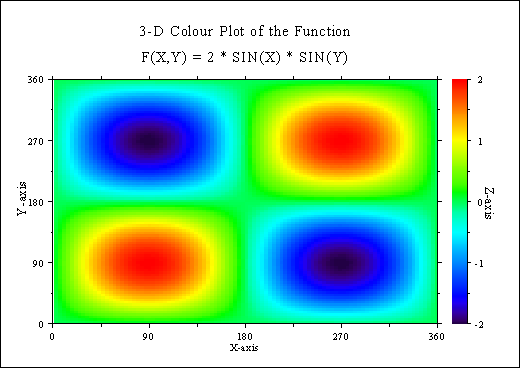## B.2 3-D Colour Plot

```%GCL
// 3-D Colour Plot

N = 100
FLOAT ZMAT[N,N], XRAY[N]

PI = 3.1415927
STEP = 2. * PI / (N - 1)

DO I = 0, N - 1
XRAY[I] = SIN (I * STEP)
END DO

DO J = 0, N - 1
ZMAT[*,J] = 2 * XRAY * SIN (J * STEP)
END DO

METAFL ('CONS')
DISINI ()
COMPLX ()
PAGERA ()

LABDIG (-1, 'XYZ')
NAME   ('X-Achse', 'X')
NAME   ('Y-Achse', 'Y')
NAME   ('Z-Achse', 'Z')
TITLIN ('3-D Colour plot of the function', 1)
TITLIN ('F(X,Y) = 2 * SIN(X) * SIN(Y)', 3)

AUTRES (50,50)
GRAF3  (0.,360.,0.,90.,0.,360.,0.,90.,-2.,2.,-2.,1.0)
TITLE  ()

CRVMAT (ZMAT, N, N, 1, 1)
MPAEPL (3)
DISFIN ()
```Figure B.2: 3-D Colour Plot

Next | Previous | Contents